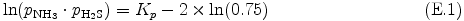## E. Initial Mixing Ratios of Condensible Volatiles

At the lower boundary, abundances of condensible elements relative to hydrogen are set to be the same as the solar abundance given by Asploud et al. (2005) . The vertical profiles of mixing ratios are obtained by the following procedures.

### H2O mixing ratio

The initial mixing ratio is homogeneous (qH2O = 7.816 × 10-4) below the level where relative humidity for the initial temperature reaches 75 %. At altitudes above that level, the mixing ratio is given as the relative humidity for the initial temperature to be 75 %.

### H2S mixing ratio

The mixing ratio is given constant as the value at the lower boundary (qH2S = 2.360 ×10-5) up to the level where the following relationship hold for the mixing ratios of H2S an NH3 given at the lower boundary with the initial temperature,At altitudes above that level, the mixing ratio is given such that eq. (E.1) will hold for the initial temperature profile. Detailed expression of Kp is given in Appendix F .

### NH3 mixing ratio

The mixing ratio is given constant as the value at the lower boundary (qNH3 = 1.030 × 10-4) below the level where eq. (E.1) is satisfied for the initial temperature and mixing ratios of H2S and NH3 at the lower boundary.

From that level up to the altitude where qH2S ≈ 0, the mixing ratio is given such that the relationship of eq. (E.1) will hold true for the initial temperature.

Then the mixing ratio is given constant above the level of qH2S ≈ 0 upto the level at which the relative humidity for the initial temperature reaches 75%.

At altitudes above this level, the mixing ratio is given such that the relative humidity for the initial temperature is 75 %.